Next: Computing the expectation of Up: Using an MDL-based cost Previous: MDL-based cost functions for

# Measuring the description length

Typically neural networks have relatively simple structure but many parameters, and it is usually easy to design a satisfactory coding for the structure. We shall therefore concentrate on measuring the description length of the parameters of the model.

If the transmitted data consists of discrete elements with known probabilities, then the length needed in transmission can be computed. It is well-known that if the probability of a data element D is P(D), then the transmission of the element D using the optimal code takes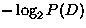bits [9,8]. We shall hereafter measure information in base e instead of base 2:bits equals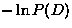nats, where 1 nat equals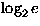bits.

The output data and the parameters in the network have continuous values instead of discrete. Communicating a random continuous value at an infinite precision would require an infinite number of bits, and therefore the values have to be discretised. If the discretisation is done with precision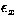, then the range of values is divided into small bins with size. Suppose we know the probability distribution p(x). Ifis small enough, p(x) is approximately linear throughout each bin and the probability of each bin is approximately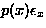, which means that the description length L of the value x is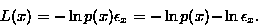(1)
Equation 1 shows that in order to compute the description length, each continuos value must be assigned a probability distribution and an accuracy.

The model is described by its structure, its parameters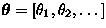and their accuracies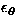. We shall consider a coding scheme where the model is used to assign a probability distribution p(D) for each data element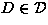. Some of the parameters may also be hyper parameters which are used to assign probability distribution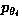for other parameters. The data is discretised with accuracy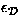, but that can be considered an integral part of the coding scheme. This is because the effect ofdoes not depend on the model: it always adds a constant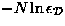term in the description length, and can therefore be omitted (N is the number of data elements). The accuracy of the parameters () does depend on the model, however, and must be taken into account.

In the literature, several different ways to measure the description length have been proposed. Our approach dates back to Wallace and Boulton  and similar approaches have been used in [4,11]. Alternative approaches have been presented e.g. in [6,7].

Suppose the target value for the parameter vector is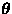. Let us denote the actual discretised value by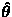and the corresponding round-off error by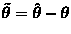. The errors depend on the locations of the borders between the bins, but there is no reason to favour one discretisation over the others, and therefore we should compute the expectation of the description length over the distribution of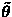,which is approximately even in the range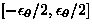. We are also going to assume that the errors are uncorrelated, that is,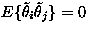.

The expected description length can be approximated by utilising the second order Taylor's series expansion of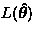about.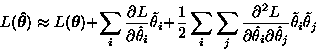(2)
Here the derivatives of L are evaluated at.Taking expectation overyields the cost function: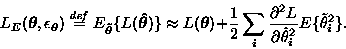(3)
Here we have used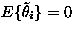for all i andfor all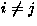. The optimal parametersand their accuraciescan be solved by minimising the expected description length LE.

Since the cost function includes second order derivatives of L with respect to the parameters, it might be troublesome to use it with a large neural network. First the outputs have to be computed in a feedforward phase as usual. Approximations of the second order derivatives needed in the cost function can be computed in a process analogous to backpropagation. It involves two feedback phases: the first one is used to compute the first order derivatives and the second one to compute the second order derivatives. It is then laborious to use backpropagation to compute the gradient, because it has to be applied to all three phases, resulting in a total of six phases.

Fortunately we can overcome these difficulties by recognising that it is not the second order derivatives we wish to evaluate but the expectation over.Next: Computing the expectation of Up: Using an MDL-based cost Previous: MDL-based cost functions for
Harri Lappalainen
5/19/1998